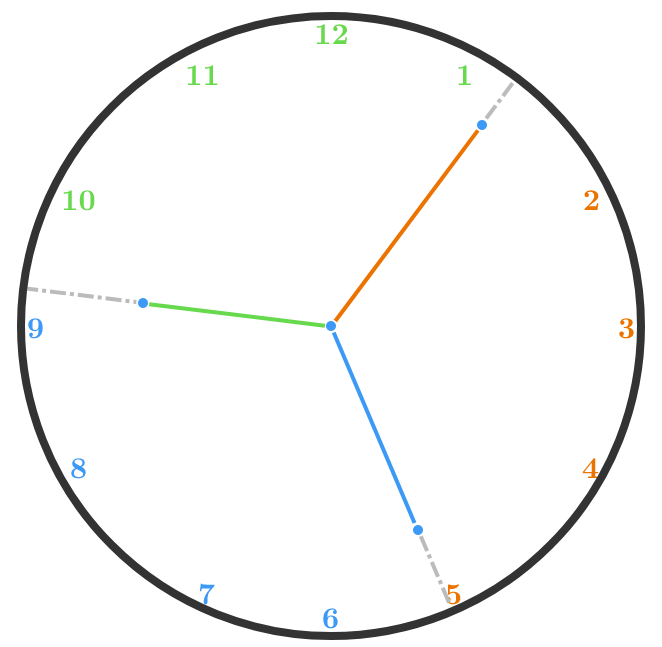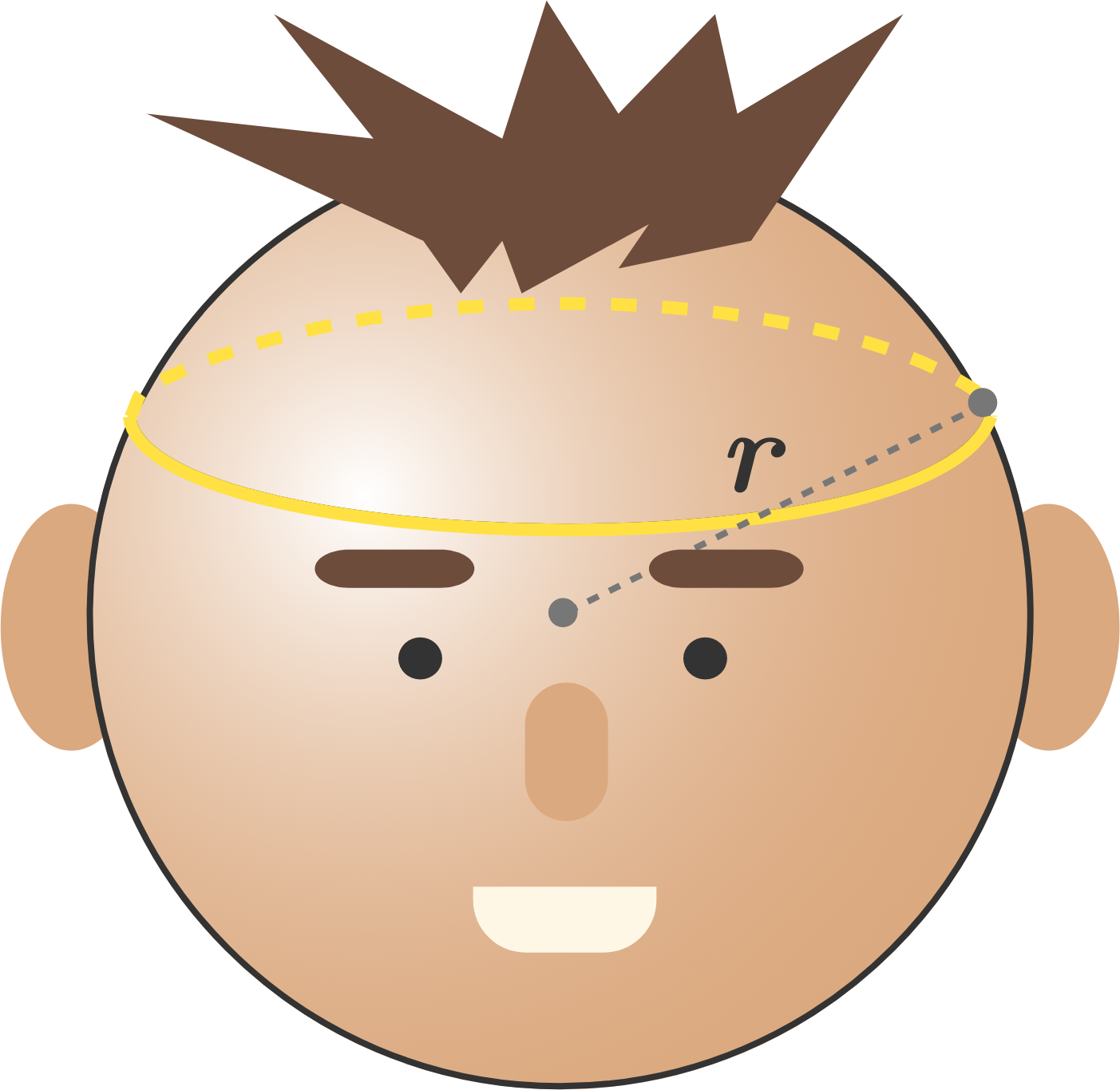# 2018-06-25 Advanced

$\underbrace{\pm (x-1) \pm (x-1) \cdots \pm (x-1) \pm (x-1)}_{“\pm (x-1)" \text{ appears 2018 times}} = 2018$

On the left side of the equation, "$\pm (x-1)$" repeats 2018 times.

How many integer solutions of $x$ are there?

Note: The "$\pm$" symbols are independent of one another.

Can the hour, minute, and second hands—when extended to the circumference—ever cut a properly functioning circular clock into three equal areas?True or False?

If $\int \frac{e^x}{1+x^2} \ dx = f(x)e^x + C,$ where $C$ is a constant, then $f$ is a rational function. That is, $f(x) = \frac{\mathrm{P}(x)}{\mathrm{Q}(x)},$ where $\mathrm{P}(x)$ and $\mathrm{Q}(x)$ are real polynomials in $x.$

Note: This problem was adapted from a question in the 2016 STEP III exam.The blacksmiths of the kingdom of Mechania are wondering how much tension is holding a crown together as it rests on the head of their sovereign. The king of Mechania has a frictionless, perfectly spherical head with radius $r = 10 \text{ cm}$, and the crown is a thin cord with a length of $\ell = 37.7 \text{ cm}$ and a mass of $m = 628.32 \text{ g}.$

Compute the tension in the crown (in Newtons).

Assumptions:

• The crown has no thickness and rests perfectly level (that is, it makes a circle which is contained in a plane parallel to the horizontal).
• The gravitational constant of Mechania is $g = 10 \text{ m/s}^2.$

We know a unit sphere will look like a unit circle when viewed from any direction.

Now, we have a solid which has the silhouette of a unit circle when viewed from the top, front, or side (from perpendicular directions).

Find the maximum volume of this solid.

×

Problem Loading...

Note Loading...

Set Loading...